International
Tables for
Crystallography
Volume A
Space-group symmetry
Edited by M. I. Aroyo

International Tables for Crystallography (2015). Vol. A, ch. 1.4, pp. 64-66

## Section 1.4.4.3. Wyckoff sets

B. Souvignierd

#### 1.4.4.3. Wyckoff sets

| top | pdf |

Points belonging to the same Wyckoff position have conjugate site-symmetry groups and thus in particular all those points are collected together that lie in one orbit under the space group. However, in addition, points that are not symmetry-related by a symmetry operation inmay still play geometrically equivalent roles, e.g. as intersections of rotation axes with certain reflection planes.

#### Example

In the conventional setting, the fourfold axes of a space groupof type P4 (75) intersect the ab plane in the points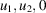and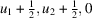for integers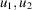, as can be seen from the space-group diagram in Fig. 1.4.4.3.Figure 1.4.4.3 | top | pdf |Symmetry-element diagram for the space group P4 (75) for the orthogonal projection along .

The pointslie in one orbit under the translation subgroup of, and thus belong to the same Wyckoff position, labelled 1a. For the same reason, the pointsbelong to a single Wyckoff position, namely to position 1b. The points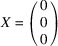and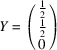do not belong to the same Wyckoff position, because the site-symmetry group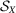is generated by the fourfold rotation 4001 and conjugating this by an operation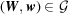results in a fourfold rotation with axis parallel to the c axis and running through w. But since the translation parts of all operations inare integral, such an axis can not containand thusand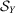are not conjugate in.

However, the translation by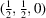conjugatesto, while fixing the groupas a whole. This shows that there is an ambiguity in choosing the origin either at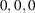or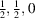, since these points are geometrically indistinguishable (both being intersections of a fourfold axis with the ab plane).

The ambiguity in the origin choice in the above example can be explained by the affine normalizer of the space group(see Section 1.1.8for a general introduction to normalizers). The full groupof affine mappings acts via conjugation on the set of space groups and the space groups of the same affine type are obtained as the orbit of a single group of that type under.

#### Definition

The groupof affine mappings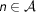that fix a space groupunder conjugation is called the affine normalizer of, i.e.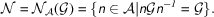The affine normalizer is the largest subgroup ofsuch thatis a normal subgroup of.

Conjugation by operations of the affine normalizer results in a permutation of the operations of, i.e. in a relabelling without changing their geometric properties. The additional translations contained in the affine normalizer can in fact be derived from the space-group diagrams, because shifting the origin by such a translation results in precisely the same diagram. More generally, an element of the affine normalizer can be interpreted as a change of the coordinate system that does not alter the space-group diagrams.

A more thorough description of the affine normalizers of space groups is given in Chapter 3.5, where tables with the affine normalizers are also provided.

Since the affine normalizer of a space groupis in general a group containingas a proper subgroup, it is possible that subgroups ofthat are not conjugate by any operation ofmay be conjugate by an operation in the affine normalizer. As a consequence, the site-symmetry groupsandof two points X and Y belonging to different Wyckoff positions ofmay be conjugate under the affine normalizer of. This reveals that the points X and Y are in fact geometrically equivalent, since they fall into the same orbit under the affine normalizer of. Joining the equivalence classes of these points into a single class results in a coarser classification with larger classes, which are called Wyckoff sets.

#### Definition

Two points X and Y belong to the same Wyckoff set if their site-symmetry groupsandare conjugate subgroups of the affine normalizer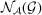of.

In particular, the Wyckoff set containing a point X also contains the full orbit of X under the affine normalizer of.

#### Example

Letbe the space group of type(17) generated by the translations of an orthorhombic lattice, the twofold rotation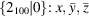and the twofold screw rotation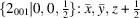. Note that the composition of these two elements is the twofold rotation with the line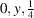as its geometric element. The grouphas four different Wyckoff positions with a site-symmetry group generated by a twofold rotation; representatives of these Wyckoff positions are the points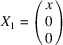(Wyckoff position 2a, site-symmetry symbol 2..),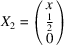(position 2b, symbol 2..),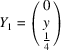(position 2c, symbol .2.) and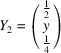(position 2d, symbol .2.).

From the tables of affine normalizers in Chapter 3.5, but also by a careful analysis of the space-group diagrams in Fig. 1.4.4.4, one deduces that the affine normalizer ofcontains the additional translations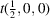,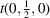and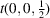, since all the diagrams are invariant by a shift ofalong any of the coordinate axes. Moreover, the symmetry operation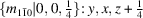which interchanges the a and b axes and shifts the origin byalong the c axis belongs to the affine normalizer, because it precisely interchanges the twofold rotations around axes parallel to the a and to the b axes. The translationmaps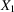to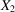, and henceandhave site-symmetry groups which are conjugate under the affine normalizer ofand thus belong to the same Wyckoff set. Analogously,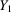and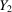belong to the same Wyckoff set, becausemapsto. Finally, the operation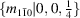found in the affine normalizer mapsto. This shows that the points of all four Wyckoff positions actually belong to the same Wyckoff set.Figure 1.4.4.4 | top | pdf |Symmetry-element diagrams for the space group(17) for orthogonal projections along , ,  (left to right).

Geometrically, the positions in this Wyckoff set can be described as those points that lie on a twofold rotation axis.

The assignments of Wyckoff positions of plane and space groups to Wyckoff sets are discussed and tabulated in Chapter 3.4.

Remark: The previous example deserves some further discussion. The groupof typebelongs to the orthorhombic crystal family, and the conventional unit cell is spanned by three basis vectors a, b, c with lengths a, b, c and right angles between each pair of basis vectors. Unless the parameters a and b are equal because of some metric specialization, the operationof the affine normalizer is not an isometry but changes lengths. If it is desired that the metric properties are preserved, the full affine normalizer cannot be taken into account, but only the subgroup that consists of isometries. This subgroup is called the Euclidean normalizer of. (A detailed discussion of Euclidean normalizers of space groups and their tabulation are given in Chapter 3.5.)

Taking conjugacy of the site-symmetry groups under the Euclidean normalizer as a condition results in a notion of equivalence which lies between that of Wyckoff positions and Wyckoff sets. In the above example, the four Wyckoff positions would be merged into two classes represented byand, butandwould not be regarded as equivalent, since they are not related by an operation of the Euclidean normalizer.

It turns out, however, that in many cases this intermediate classification coincides with the Wyckoff sets, because points belonging to different Wyckoff positions are often related to each other by a translation contained in the affine normalizer. Since translations are always isometries, the translations contained in the affine normalizer always belong to the Euclidean normalizer as well.